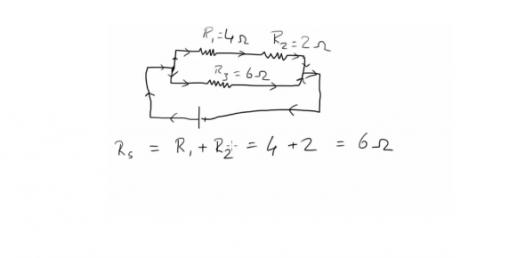# Quiz: 10th Grade Physics Questions!

5 Questions | Total Attempts: 2694Settings.

• 1.
The magnification produced by a mirror is 1/3, then the type of mirror is _____
• A.

Concave

• B.

Convex

• C.

Plane

• 2.
An electric current through a metallic conductor produces _________ around it.
• A.

Heat

• B.

Light

• C.

Magnetic field magnetic field

• D.

Mechanical force

• 3.
The field of view is maximum for ______
• A.

Plane mirror

• B.

Concave mirror

• C.

Convex mirror

• 4.
An object is placed 25 cm from a convex lens whose focal length is 10 cm. The image distance is ________
• A.

50 cm

• B.

16.66 cm

• C.

6.66 cm

• D.

10 cm

• 5.
From the following statement write down that which is applicable to a commutator.
• A.